# The ARIMAX model muddle

October 4, 2010
By

Want to share your content on R-bloggers? click here if you have a blog, or here if you don't.

There is often confusion about how to include covariates in ARIMA models, and the presentation of the subject in various textbooks and in R help files has not helped the confusion. So I thought I’d give my take on the issue. To keep it simple, I will only describe non-seasonal ARIMA models although the ideas are easily extended to include seasonal terms. I will include only one covariate in the models although it is easy to extend the results to multiple covariates. And, to start with, I will  assume the data are stationary, so we only consider ARMA models.

Let the time series be denoted by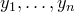. First, we will define an ARMAmodel with no covariates:whereis a white noise process (i.e., zero mean and iid).

#### ARMAX models

An ARMAX model simply adds in the covariate on the right hand side:whereis a covariate at timeandis its coefficient. While this looks straight-forward, one disadvantage is that the covariate coefficient is hard to interpret. The value ofis not the effect onwhen theis increased by one (as it is in regression). The presence of lagged values of the response variable on the right hand side of the equation mean thatcan only be interpreted conditional on the value of previous values of the response variable, which is hardly intuitive.

If we write the model using backshift operators, the ARMAX model is given by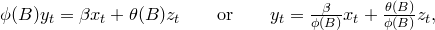qquadtext{or}qquad
y_t = frac{beta}{phi(B)}x_t + frac{theta(B)}{phi(B)}z_t,
” title=” phi(B)y_t = beta x_t + theta(B)z_t
y_t = frac{beta}{phi(B)}x_t + frac{theta(B)}{phi(B)}z_t,
” style=”vertical-align: -10px; border: none;”/>

whereand.
Notice how the AR coefficients get mixed up with both the covariates and the error term.

#### Regression with ARMA errors

For this reason, I prefer to use regression models with ARMA errors, defined as follows.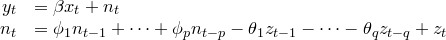y_t &= beta x_t + n_t\
n_t &= phi_1 n_{t-1} + cdots + phi_p n_{t-p} – theta_1 z_{t-1} – dots – theta_q z_{t-q} + z_t
end{array}” title=”begin{array}{rl}
y_t &= beta x_t + n_t\
n_t &= phi_1 n_{t-1} + cdots + phi_p n_{t-p} – theta_1 z_{t-1} – dots – theta_q z_{t-q} + z_t
end{array}” style=”vertical-align: -17px; border: none;”/>

In this case, the regression coefficient has its usual interpretation. There is not much to choose between the models in terms of forecasting ability, but the additional ease of interpretation in the second one makes it attractive.

Using backshift operators, this model can be written as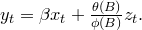y_t = beta x_t + frac{theta(B)}{phi(B)}z_t.” title=”
y_t = beta x_t + frac{theta(B)}{phi(B)}z_t.” style=”vertical-align: -10px; border: none;”/>

Transfer function models

Both of these models can be considered as special cases of transfer function models, popularized by Box and Jenkins: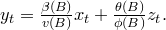This allows for lagged effects of covariates (via theoperator) and for decaying effects of covariates (via theoperator).

Sometimes these are called “dynamic regression models”, although different books use that term for different models.

The method for selecting the orders of a transfer function model that is described in Box and Jenkins is cumbersome and difficult, but continues to be described in textbooks. A much better procedure is given in Pankratz (1991), and repeated in my 1998 forecasting textbook.

#### Non-stationary data

For ARIMA errors, we simply replacewith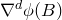where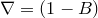denotes the differencing operator. Notice that this is equivalent to differencing bothandbefore fitting the model with ARMA errors. In fact, it is necessary to difference all variables first as estimation of a model with non-stationary errors is not consistent and can lead to “spurious regression”.

#### R functions

The `arima()` function in R (and `Arima()` and `auto.arima()` from the forecast package) fits a regression with ARIMA errors. Unfortunately there is a bug in the code so that if a non-stationary error is specified, the data are not differenced before estimation of the regression coefficients. Consequently, you should do your own differencing when required. Note also that R reverses the signs of the moving average coefficients compared to the standard parameterization given above.

The `arimax()` function from the TSA package fits the transfer function model (but not the ARIMAX model). This is a new package and I have not yet used it, but it is nice to finally be able to fit transfer function models in R. Sometime I plan to write a function to allow automated order selection for transfer functions as I have done with `auto.arima()` for regression with ARMA errors (part of the forecast package)

R-bloggers.com offers daily e-mail updates about R news and tutorials about learning R and many other topics. Click here if you're looking to post or find an R/data-science job.
Want to share your content on R-bloggers? click here if you have a blog, or here if you don't.

Tags: , , ,